# 1.学习总结

## 1.1查找的思维导图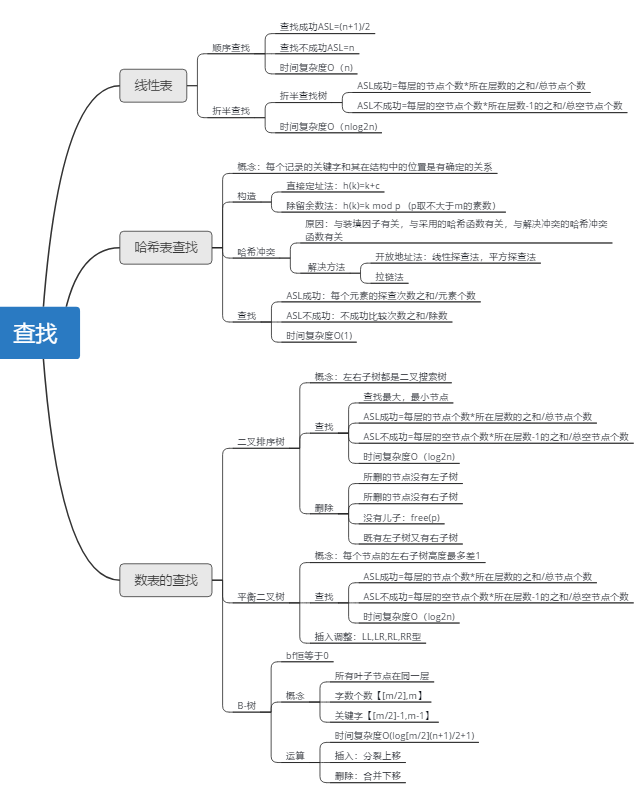## 1.2 查找学习体会

• 在数据结构中查找是一种很常见的结构
• 二叉搜索树、平衡二叉树、AVL树、B-树、B+树，各种查找树都有各自的ASL算法

# 2.PTA实验作业

## 题目1：是否二叉搜索树

### 设计思路：

   用中序遍历来遍历二叉树
再将中序遍历收集到一个数组里
判断数组是否为从小到大排序
if 是则为二叉搜索树
else 不是二叉搜索树


### 代码截图：### PTA提交列表说明：## 题目2：二叉搜索树中的最近公共祖先

### 设计思路：


先判断两个数值是否在二叉树内
if没有则返回ERROR
else
if 一个在节点的左子树里，一个在节点的右子树里  返回节点
if 都在左子树，递归左子树
if 都在右子树，递归右子树


### 代码截图：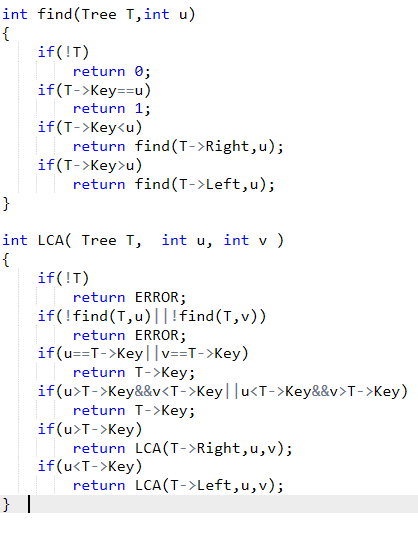### PTA提交列表说明：## 题目3：QQ帐户的申请与登陆

### 设计思路：

   定义map迭代器
输入类型 QQ号 密码
if（N）
在容器中查找，if找到，则申请失败
else 申请成功 并将该数据插入迭代器中
if(L)
在容器中查找，if 找到，则登入成功
else 没找到不匹配，登入失败


### 代码截图：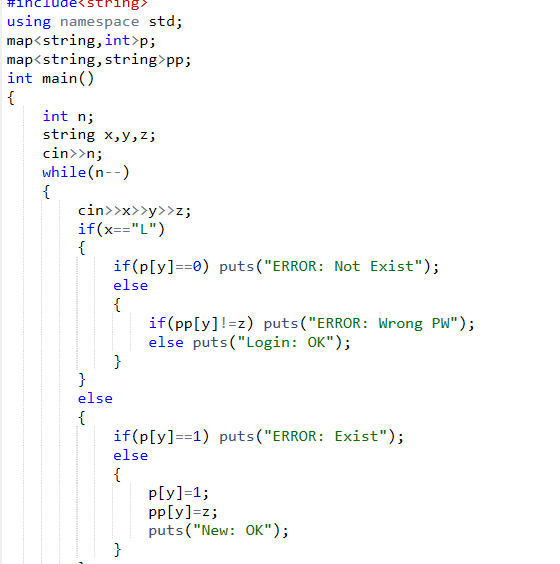### PTA提交列表说明：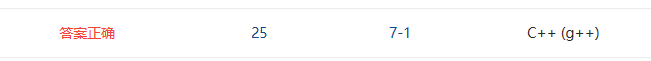# 3.截图本周题目集的PTA最后排名

## 3.1 PTA排名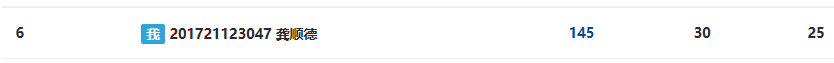# 4. 阅读代码

#include <cstdio>//基于词频的文件相似度
#include <cstring>
#include <algorithm>
#include <set>
#include <string>
using namespace std;

int main(int argc, char const *argv[]) {
int n, m, a, b;
char s, tmp;
set<string> st;
scanf("%d%*c", &n);
for(int i=1; i<=n; ++i) {
while(gets(s)) {
if(!strcmp(s, "#")) break;
int last = 0;
for(int j=0; s[j]; ++j) {
if(s[j]>='A' && s[j]<='Z') s[j] += 32;
// 分割单词，符合要求的存进 set
if(s[j]<'a' || s[j]>'z') {
s[j] = 0;
strcpy(tmp, s+last);
last = j+1;
tmp = 0;
if(strlen(tmp) >= 3) st[i].insert(tmp);
}
}
strcpy(tmp, s+last);
tmp = 0;
if(strlen(tmp) >= 3) st[i].insert(tmp);
}
}
scanf("%d", &m);
while(m--) {
scanf("%d %d", &a, &b);
int cnt = 0;
set<string>::iterator it;
// !important: 遍历 size 较小的 set
if(st[a].size() > st[b].size()) swap(a, b);
for(it=st[a].begin(); it!=st[a].end(); ++it) {
if(st[b].count(*it)) cnt++;
}
printf("%.1f%%\n", 100.0*cnt/(st[a].size()+st[b].size()-cnt));
}
return 0;
}


# 5. 代码Git提交记录截图

posted on 2018-05-26 21:27  ifcan  阅读(336)  评论(0编辑  收藏  举报